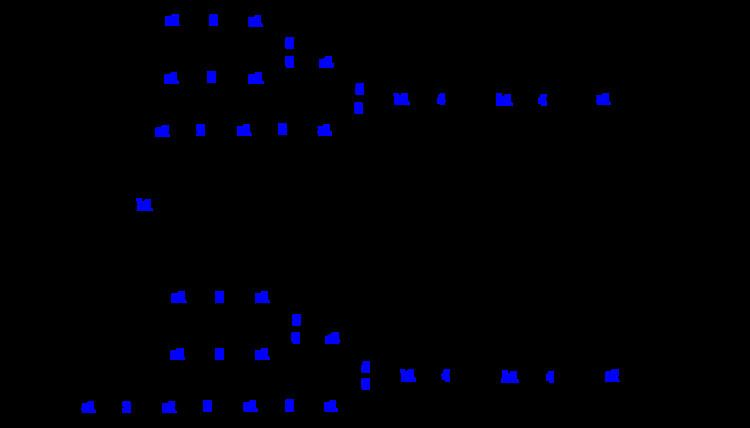ALG6 (enzyme class)

Updated on
 EC number  2.4.1.267BRENDA  BRENDA entryKEGG  KEGG entry IntEnz  IntEnz viewExPASy  NiceZyme viewMetaCyc  metabolic pathwayDolichyl-P-Glc:Man9GlcNAc2-PP-dolichol alpha-1,3-glucosyltransferase (EC 2.4.1.267, ALG6, Dol-P-Glc:Man9GlcNAc2-PP-Dol alpha-1,3-glucosyltransferase) is an enzyme with systematic name dolichyl beta-D-glucosyl phosphate:D-Man-alpha-(1->2)-D-Man-alpha-(1->2)-D-Man-alpha-(1->3)-(D-Man-alpha-(1->2)-D-Man-alpha-(1->3)-(D-Man-alpha-(1->2)-D-Man-alpha-(1->6))-D-Man-alpha-(1->6))-D-Man-beta-(1->4)-D-GlcNAc-beta-(1->4)-D-GlcNAc-diphosphodolichol alpha-1,3-glucosyltransferase.

This enzyme catalyses the following chemical reaction

which is:

dolichyl beta-D-glucosyl phosphate + D-Man-alpha-(1->2)-D-Man-alpha-(1->2)-D-Man-alpha-(1->3)-[D-Man-alpha-(1->2)-D-Man-alpha-(1->3)-[D-Man-alpha-(1->2)-D-Man-alpha-(1->6)]-D-Man-alpha-(1->6)]-D-Man-beta-(1->4)-D-GlcNAc-beta-(1->4)-D-GlcNAc-diphosphodolichol D-Glc-alpha-(1->3)-D-Man-alpha-(1->2)-D-Man-alpha-(1->2)-D-Man-alpha-(1->3)-[D-Man-alpha-(1->2)-D-Man-alpha-(1->3)-[D-Man-alpha-(1->2)-D-Man-alpha-(1->6)]-D-Man-alpha-(1->6)]-D-Man-beta-(1->4)-D-GlcNAc-beta-(1->4)-D-GlcNAc-diphosphodolichol + dolichyl phosphate

Topics